# Multi-level Latent Class Analysis with {MplusAutomation} ~ ~ ~

## A tutorial replicating the models presented in Henry & Muthén (2010) • LCA with nested data • 2-level models •

### 2/7/2020

Note:

• The example used in this tutorial, data about teacher discipline, is different from the example presented in Henry & Muthén (2010). This data is not currently publicly available.
• All models are estimated in `Mplus` via the R package `MplusAutomation`.

### Prepare & explore data

``````library(tidyverse)
library(haven)
library(MplusAutomation)
library(rhdf5)
library(here)
library(glue)
library(stargazer)
library(gt)
library(janitor)
library(semPlot)
library(reshape2)
library(cowplot)``````

``````data_spss <- read_spss(here("data", "teacher_discip_strat_data.sav")) %>%
clean_names()

# write a CSV datafile (to remove labels)
write_csv(data_spss, here("data", "teach_discip_data.csv"))``````

read the unlabeled data back into R

``mlca_data <- read_csv(here("data", "teach_discip_data.csv"), na = c("9999"))``

view labeled data (create a codebook)

``sjPlot::view_df(data_spss)``

prepare data for `MplusAutomation`

``````mlca_mplus <- mlca_data %>%
select(-id, -districtname, -schoolname) # remove columns with strings``````

shorten names to be `< 8` characters

``````names(mlca_mplus) <-  str_remove(names(mlca_mplus), pattern = "itive")

mlca_mplus <- mlca_mplus %>%
rename(pop =  population,  # Bullying is a big problem in this school
distcode = districtcode,
schlcode = schoolcode,
postcode = positioncode)``````

View descriptive statistics for LCA measurement indicators

``````lca_summary <- mlca_mplus %>%
select(53:67)

Make table grouped by school (`schlcode`)

``````# how many school clusters are there?
# length(unique(mlca_mplus\$schlcode)) # 130 schools

school_summary <- mlca_mplus %>%
group_by(schlcode) %>%
summarize(
mean_lvl = mean(level, na.rm = TRUE),
mean_pun_1 = mean(pun_1, na.rm = TRUE),
mean_pos_1 = mean(pos_1, na.rm = TRUE),
mean_sel_1 = mean(sel_1, na.rm = TRUE),
sample_n = n())

school_summary[1:10,] %>%
gt() ``````
schlcode mean_lvl mean_pun_1 mean_pos_1 mean_sel_1 sample_n
10 1 1.704545 3.545455 3.477273 44
11 1 1.482759 3.482759 3.413793 29
14 1 1.451613 3.483871 3.548387 31
16 2 1.794872 3.102564 3.000000 39
17 2 1.797101 3.260870 3.043478 69
18 3 1.871795 2.948718 2.833333 78
20 1 1.435897 3.615385 3.615385 39
21 1 1.659574 3.617021 3.319149 47
23 2 1.838710 3.096774 3.032258 31
24 3 1.825000 2.825000 2.375000 40

Note: In order to reduce estimation time for this example 7 indicators were chosen and dichotomized. For the same reason the 4-class solution was used in all MLCA models for purposes of demonstration.

### Recode data

7 indicators used in LCA demonstration

1. pos_1 = Students are praised often.
2. pos_3 = Teachers often let students know when they are being good.
3. pos_2 = Students are often given rewards for being good.
4. pos_4 = Classes get rewards for good 1 behavior.
5. sel_5 = Students are taught they should care about how others feel.
6. sel_2 = Students are taught to understand how others think and feel.
7. sel_1 = Students are taught to feel responsible for how they act.

convert indicators to be dichotomous

``````mlca_mplus <- mlca_mplus %>%
mutate(
pos_1b = case_when(
pos_1 <  3 ~ 0,        # disagree ~ responses 1 & 2
pos_1 >= 3 ~ 1)) %>%   # agree ~ responses 3 & 4
mutate(
pos_3b = case_when(
pos_3 <  3 ~ 0,
pos_3 >= 3 ~ 1)) %>%
mutate(
pos_2b = case_when(
pos_2 <  3 ~ 0,
pos_2 >= 3 ~ 1)) %>%
mutate(
pos_4b = case_when(
pos_4 <  3 ~ 0,
pos_4 >= 3 ~ 1)) %>%
mutate(
sel_5b = case_when(
sel_5 <  3 ~ 0,
sel_5 >= 3 ~ 1)) %>%
mutate(
sel_2b = case_when(
sel_2 <  3 ~ 0,
sel_2 >= 3 ~ 1)) %>%
mutate(
sel_1b = case_when(
sel_1 <  3 ~ 0,
sel_1 >= 3 ~ 1))

table(mlca_mplus\$sel_1)``````
``````##
##    1    2    3    4
##   81  659 3190 1157``````
``table(mlca_mplus\$sel_1b)``
``````##
##    0    1
##  740 4347``````

### model 00: LCA enumeration (fixed effect model)

``````lca_k1_6  <- lapply(1:6, function(k) {
lca_enum  <- mplusObject(

TITLE = glue("C{k}_mlca_enum_demo"),

VARIABLE =
glue(
"categorical = pos_1b-sel_1b;
usevar = pos_1b-sel_1b;

classes = c({k});"),

ANALYSIS =
"estimator = mlr;
type = mixture;
starts = 500 100;",

MODEL = "",
OUTPUT = "",

PLOT =
"type = plot3;
series = pos_1b-sel_1b(*);",

usevariables = colnames(mlca_mplus),
rdata = mlca_mplus)

lca_enum_fit <- mplusModeler(lca_enum,
dataout=glue(here("enum_mplus", "c_{k}_mlca_enum.dat")),
modelout=glue(here("enum_mplus", "c_{k}_mlca_enum.inp")) ,
check=TRUE, run = TRUE, hashfilename = FALSE)
})``````

### Read models & plot LCA (\(K=4\))

``````output_enum <- readModels(here("enum_mplus"), quiet = TRUE)

enum_summary <- LatexSummaryTable(output_enum,
keepCols=c("Title",
"LL",
"BIC",
"aBIC"),
sortBy = "Title")

enum_summary %>%
gt()``````
Title LL BIC aBIC
C1_mlca_enum_demo -13867.62 27794.98 27772.73
C2_mlca_enum_demo -11348.33 22824.67 22777.01
C3_mlca_enum_demo -10971.08 22138.45 22065.36
C4_mlca_enum_demo -10671.22 21607.00 21508.50
C5_mlca_enum_demo -10628.89 21590.62 21466.69
C6_mlca_enum_demo -10600.19 21601.50 21452.15

plot 4-class LCA probability plot

``````# extract posterior probabilities
pp1 <- as.data.frame(output_enum[["c_4_mlca_enum.out"]]
[["gh5"]]
[["means_and_variances_data"]]
[["estimated_probs"]]
[["values"]]
[seq(2, 14, 2),]) #seq("from","to","by")

# extract model estimated class sizes
c_size <- as.data.frame(output_enum[["c_4_mlca_enum.out"]]
[["class_counts"]]
[["modelEstimated"]]
[["proportion"]])

colnames(c_size) <- paste0("cs")
c_size <- c_size %>% mutate(cs = round(cs*100, 1))

colnames(pp1) <- paste0("C", 1:4, glue(" ({c_size[1:4,]}%)"))
pp1 <- cbind(Var = paste0("U", 1:7), pp1)

# choose the order of indicators & label
pp1\$Var <- factor(pp1\$Var,
levels = c("U1","U2","U3","U4","U5", "U6", "U7"),
labels = c("Verbal2", "Verbal1", "Reward1", "Reward2",
"Empathy1","Empathy2", "Responsible"))

pd_long <- melt(pp1, id.vars = "Var")

# plot data
ggplot(pd_long, aes(as.integer(Var), value, shape = variable,
colour = variable, lty = variable)) +
geom_point(size = 4) + geom_line() +
scale_x_continuous("", breaks = 1:7, labels = pp1\$Var) +
scale_y_continuous("Probability") +
scale_colour_grey() +
theme_cowplot() +
theme(text=element_text(family="Times New Roman", size=12),
legend.key.width = unit(.5, "line"),
legend.text = element_text(family="Times New Roman", size=12),
legend.title = element_blank(),
legend.position = "top")``````
``ggsave(here("figures","C4_LCA_MLCA.png"), dpi=300, height=5, width=8, units="in")``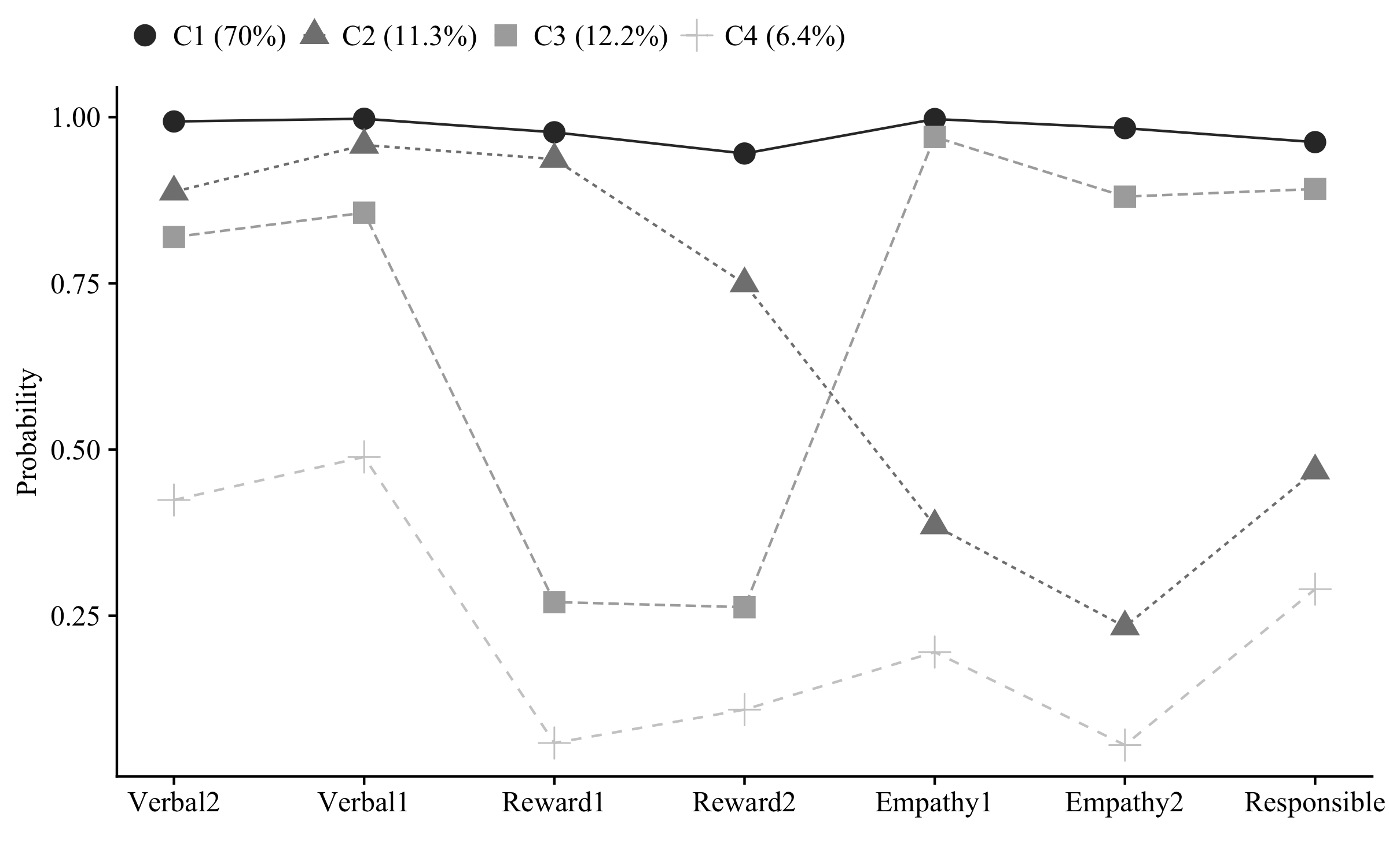### model00: Compute intra-class correlations (type = basic; w/ analysis = TWOLEVEL;)

``````# Note: In this example the ICC's are zero because items are dichotomous

mlca_00  <- mplusObject(

TITLE = "model00_basic__ICC_mlca",

VARIABLE =
"usevar = pos_1b-sel_1b;

cluster = schlcode;
within = pos_1b-sel_1b;",

ANALYSIS =
"estimator = mlr;
type = basic twolevel; ! ask for ICC curves
processors = 10;",

MODEL = "",

OUTPUT = "sampstat;",

PLOT = "",

usevariables = colnames(mlca_mplus),
rdata = mlca_mplus)

mlca_00_fit <- mplusModeler(mlca_00,
dataout=here("mlca_mplus", "model00_basic.dat"),
modelout=here("mlca_mplus", "model00_basic.inp"),
check=TRUE, run = TRUE, hashfilename = FALSE)``````

### model01: parametric random effects model (4-class)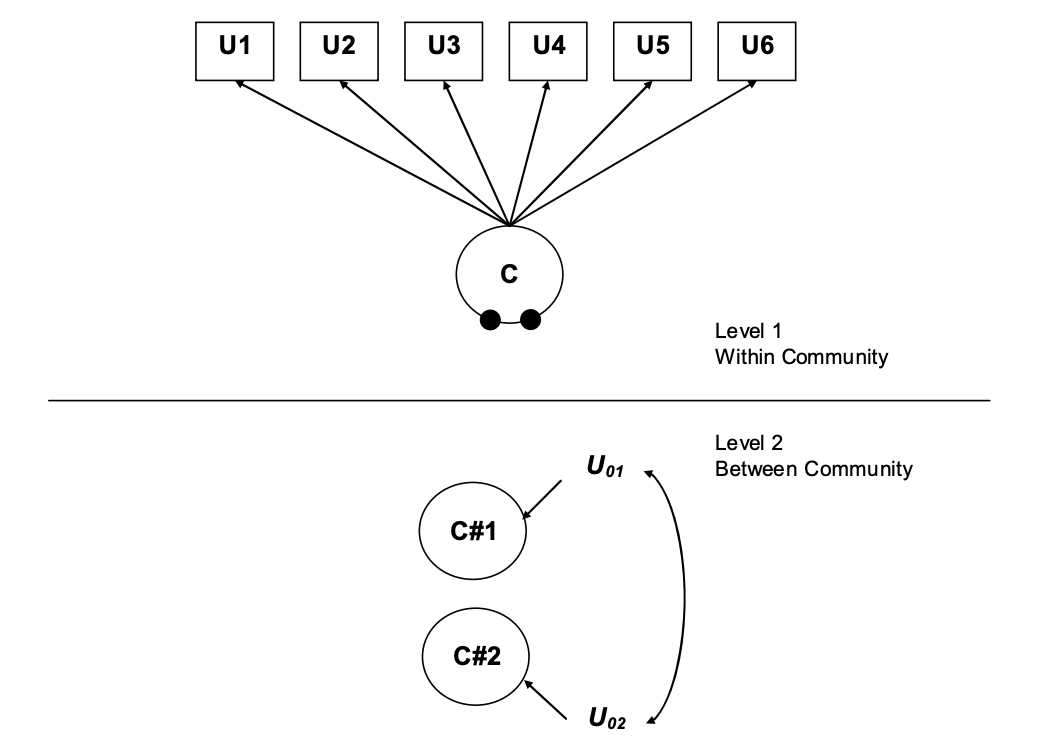Figure 1. Picture adapted from, Henry & Muthen 2010

``````# warning, run-time is very slow

mlca_01  <- mplusObject(

TITLE = "model01_parametric_mlca",

VARIABLE =
"usevar = pos_1b-sel_1b;
categorical = pos_1b-sel_1b;
classes = c(4);

cluster = schlcode;      ! level 2 units are schools
within = pos_1b-sel_1b;",

ANALYSIS =
"estimator = mlr;
type = mixture twolevel;
integration=montecarlo(1000);
starts = 100 50;
processors = 10;",

MODEL =
"%WITHIN%
%OVERALL%

%BETWEEN%
%OVERALL%
C#1;
C#2;
C#3;
C#1 WITH C#2;
C#3 WITH C#1 C#2; ",

OUTPUT = "TECH8;",

PLOT =
"type = plot3;
series = pos_1b-sel_1b(*);",

usevariables = colnames(mlca_mplus),
rdata = mlca_mplus)

mlca_01_fit <- mplusModeler(mlca_01,
dataout=here("mlca_mplus", "model01_parametric.dat"),
modelout=here("mlca_mplus", "model01_parametric.inp"),
check=TRUE, run = FALSE, hashfilename = FALSE)``````

### model02: parametric model with 2nd level factor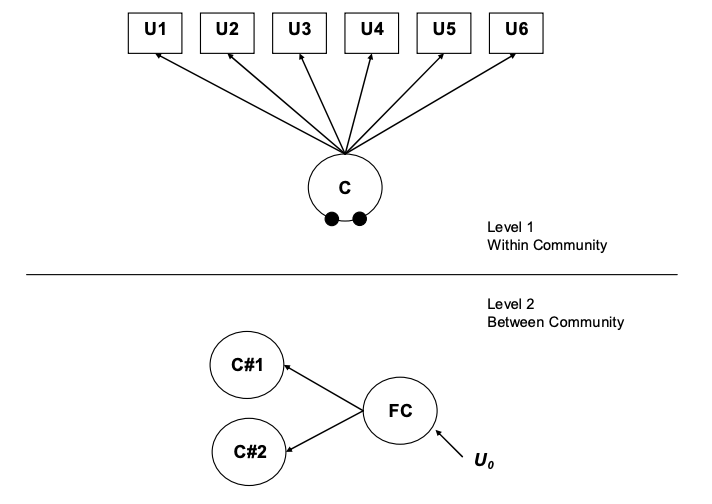Figure 2. Picture adapted from, Henry & Muthen 2010

``````  mlca_02  <- mplusObject(

TITLE = "model02_parametric_mlca",

VARIABLE =
"usevar = pos_1b-sel_1b;
categorical = pos_1b-sel_1b;
classes = c(4);

cluster = schlcode;      ! level 2 units are schools
within = pos_1b-sel_1b;",

ANALYSIS =
"estimator = mlr;
type = mixture twolevel;
starts = 20 10;
processors = 10;",

MODEL =
"%WITHIN%
%OVERALL%

%BETWEEN%
%OVERALL%
FC by C#1 C#2 C#3;",

OUTPUT = "TECH8;",

PLOT =
"type = plot3;
series = pos_1b-sel_1b(*);",

usevariables = colnames(mlca_mplus),
rdata = mlca_mplus)

mlca_02_fit <- mplusModeler(mlca_02,
dataout=here("mlca_mplus", "model02_parametric.dat"),
modelout=here("mlca_mplus", "model02_parametric.inp"),
check=TRUE, run = FALSE, hashfilename = FALSE)``````

### model03: non-parametric model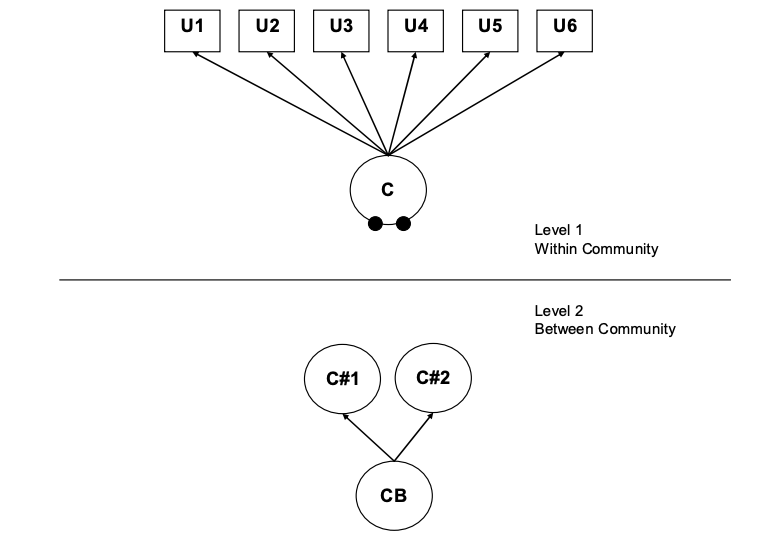Figure 3. Picture adapted from, Henry & Muthen 2010

``````  mlca_03  <- mplusObject(

TITLE = "model03_non_parametric_mlca",

VARIABLE =
"usevar = pos_1b-sel_1b;
categorical = pos_1b-sel_1b;
classes = CB(3) c(4);

cluster = schlcode;      ! level 2 units are schools
within = pos_1b-sel_1b;
between = CB;",

ANALYSIS =
"estimator = mlr;
type = mixture twolevel;
starts = 20 10;
processors = 10;",

MODEL =
"%WITHIN%
%OVERALL%

%BETWEEN%
%OVERALL%
C on CB;

MODEL C:
%WITHIN%
%C#1%
[pos_1b\$1-sel_1b\$1];
%C#2%
[pos_1b\$1-sel_1b\$1];
%C#3%
[pos_1b\$1-sel_1b\$1];
%C#4%
[pos_1b\$1-sel_1b\$1]; ",

OUTPUT = "TECH8;",

PLOT =
"type = plot3;
series = pos_1b-sel_1b(*);",

usevariables = colnames(mlca_mplus),
rdata = mlca_mplus)

mlca_03_fit <- mplusModeler(mlca_03,
dataout=here("mlca_mplus", "model03_non_parametric.dat"),
modelout=here("mlca_mplus", "model03_non_parametric.inp"),
check=TRUE, run = FALSE, hashfilename = FALSE)``````

### model04: parametric model with 2nd level factor on random latent class indicators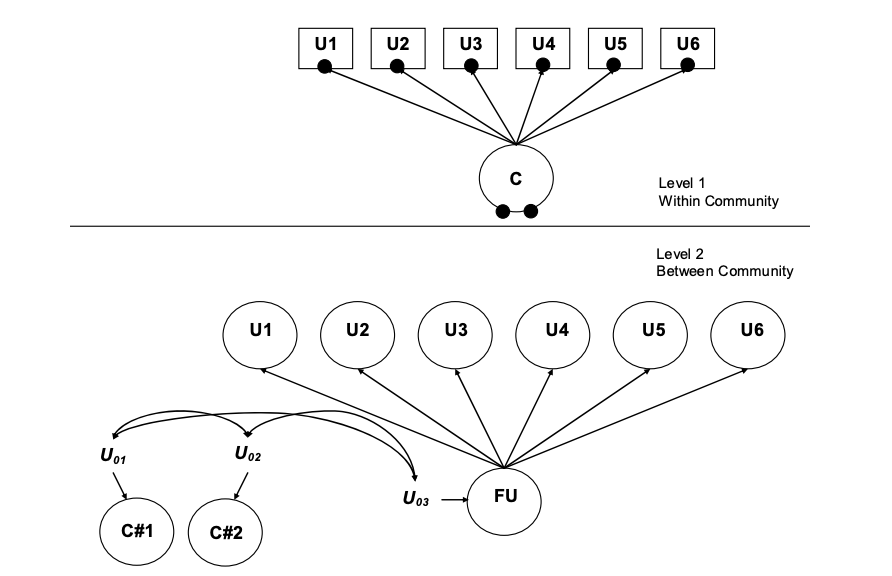Figure 4. Picture adapted from, Henry & Muthen 2010

``````  mlca_04  <- mplusObject(

TITLE = "model04_parametric_mlca",

VARIABLE =
"usevar = pos_1b-sel_1b;
categorical = pos_1b-sel_1b;
classes = c(4);

cluster = schlcode; ",

ANALYSIS =
"estimator = mlr;
type = mixture twolevel;
starts = 20 10;
processors = 10;",

MODEL =
"%WITHIN%
%OVERALL%

%BETWEEN%
%OVERALL%
FU by pos_1b-sel_1b;
[FU@0];
FU WITH C#1 C#2 C#3;
C#1;
C#2;
C#3;
C#1 WITH C#2;
C#3 WITH C#1 C#2;

%C#1%
[pos_1b\$1-sel_1b\$1];
%C#2%
[pos_1b\$1-sel_1b\$1];
%C#3%
[pos_1b\$1-sel_1b\$1];
%C#4%
[pos_1b\$1-sel_1b\$1]; ",

OUTPUT = "TECH8;",

PLOT =
"type = plot3;
series = pos_1b-sel_1b(*);",

usevariables = colnames(mlca_mplus),
rdata = mlca_mplus)

mlca_04_fit <- mplusModeler(mlca_04,
dataout=here("mlca_mplus", "model04_parametric.dat"),
modelout=here("mlca_mplus", "model04_parametric.inp"),
check=TRUE, run = F, hashfilename = FALSE)``````

### model05: parametric model with 2nd level factor on random latent class intercepts & 2nd level factor on random latent class indicators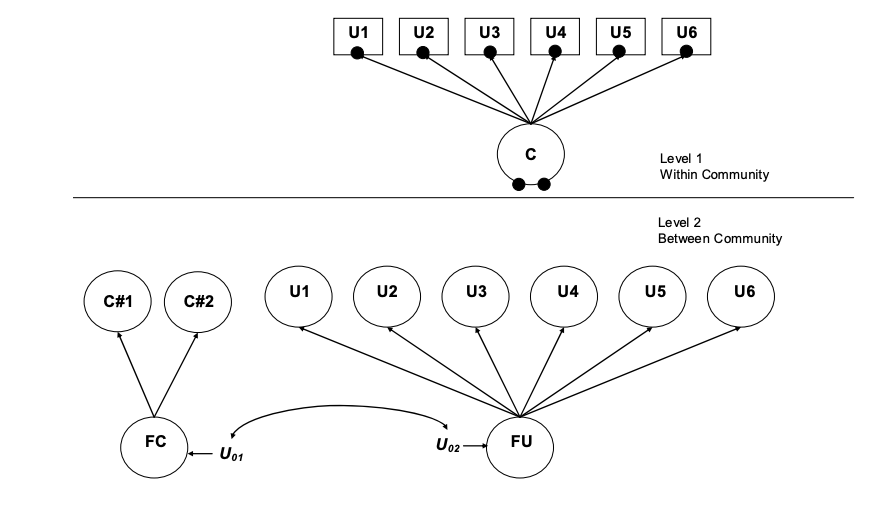Figure 5. Picture adapted from, Henry & Muthen 2010

``````  mlca_05  <- mplusObject(

TITLE = "model05_parametric_mlca",

VARIABLE =
"usevar = pos_1b-sel_1b;
categorical = pos_1b-sel_1b;
classes = c(4);

cluster = schlcode; ",

ANALYSIS =
"estimator = mlr;
type = mixture twolevel;
starts = 20 10;
processors = 10;",

MODEL =
"%WITHIN%
%OVERALL%

%BETWEEN%
%OVERALL%
FU by pos_1b-sel_1b;
[FU@0];
FC BY C#1 C#2 C#3;
FC WITH FU;

%C#1%
[pos_1b\$1-sel_1b\$1];
%C#2%
[pos_1b\$1-sel_1b\$1];
%C#3%
[pos_1b\$1-sel_1b\$1];
%C#4%
[pos_1b\$1-sel_1b\$1]; ",

OUTPUT = "TECH8;",

PLOT =
"type = plot3;
series = pos_1b-sel_1b(*);",

usevariables = colnames(mlca_mplus),
rdata = mlca_mplus)

mlca_05_fit <- mplusModeler(mlca_05,
dataout=here("mlca_mplus", "model05_parametric.dat"),
modelout=here("mlca_mplus", "model05_parametric.inp"),
check=TRUE, run = F, hashfilename = FALSE)``````

### model06: non-parametric model with level-2 factor on latent class indicators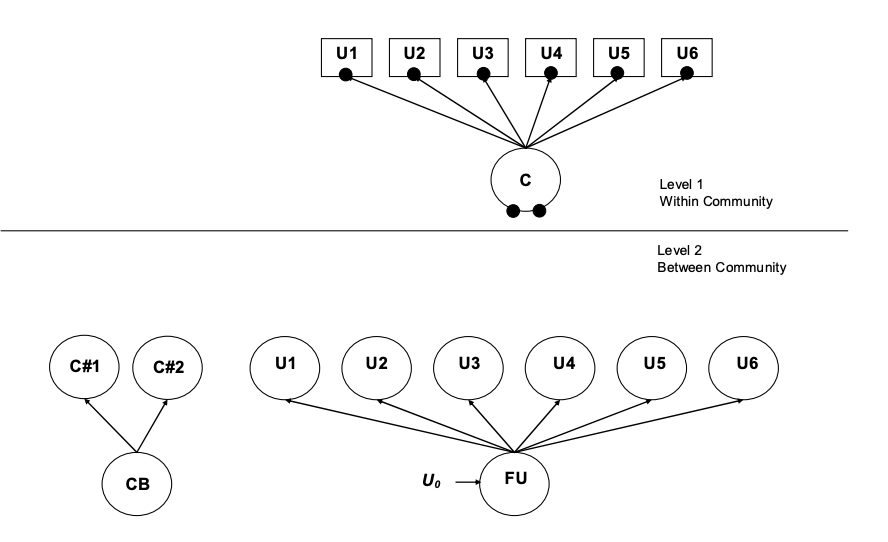Figure 6. Picture adapted from, Henry & Muthen 2010

``````  mlca_06  <- mplusObject(

TITLE = "model06_non_parametric_mlca",

VARIABLE =
"usevar = pos_1b-sel_1b;
categorical = pos_1b-sel_1b;
classes = CB(2) c(4);

cluster = schlcode;      ! level 2 units are schools
between = CB;",

ANALYSIS =
"estimator = mlr;
type = mixture twolevel;
starts = 20 10;
processors = 10;",

MODEL =
"%WITHIN%
%OVERALL%

%BETWEEN%
%OVERALL%
FU BY pos_1b-sel_1b;
[FU@0];
C on CB;

MODEL CB:
%BETWEEN%
%CB#1%
[FU@0];
%CB#2%
[FU];

MODEL C:
%BETWEEN%
%C#1%
[pos_1b\$1-sel_1b\$1];
%C#2%
[pos_1b\$1-sel_1b\$1];
%C#3%
[pos_1b\$1-sel_1b\$1];
%C#4%
[pos_1b\$1-sel_1b\$1]; ",

OUTPUT = "TECH8;",

PLOT =
"type = plot3;
series = pos_1b-sel_1b(*);",

usevariables = colnames(mlca_mplus),
rdata = mlca_mplus)

mlca_06_fit <- mplusModeler(mlca_06,
dataout=here("mlca_mplus", "model06_non_parametric.dat"),
modelout=here("mlca_mplus", "model06_non_parametric.inp"),
check=TRUE, run = FALSE, hashfilename = FALSE)``````

### model07: parametric model with 2nd level factor on random latent class intercepts & 2nd level factor on random latent class indicators

Auxiliaries: one individual-level covariate & two school-level covariates